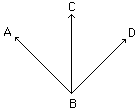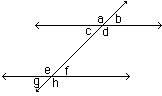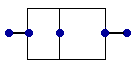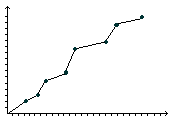Math Central - mathcentral.uregina.caResource Room— glossaries Resource RoomMathematics Glossary - Middle Years
Maxine Stinka, Saskatchewan Education, Curriculum and Instruction

Note: The definitions included here are those that are used in the Saskatchewan Education document "Mathematics 6-9: A Curriculum Guide for the Middle Level".

Various mathematics dictionaries may have different definitions. These definitions are designed to be meaningful to middle level mathematics teachers.

Select the first letter of the term that you wish to look for.
A | B | C | D | E | F | H | I | J | K | L | M | N | O | P | Q | R | S | T | U | V | W | X | Y | Z

A
• the first element in a coordinate pair. When graphed in the coordinate plane, it is the distance from the y-axis. Frequently called the x coordinate.

• an angle whose measure is between 0o and 90o.

acute triangle

• two numbers whose sum is 0.

• two angles that share a vertex and a common side between them but have no interior points in common.
•< ABC and < CBD are adjacent.

algorithm

• a step-by-step procedure for carrying out computation.

alternate angles

• two angles that are in opposite locations when lines are cut by a transversal. If the angles are between the lines, they are called alternate interior angles; if they are outside the lines, they are called alternate exterior angles. If the lines are parallel, the alternate angles are congruent.
•  Alternate interior c and f d and e Alternate exterior a and h b and g• length from the uppermost point of a triangle to the line opposite.

associative property

• when performing an operation on three or more numbers, the result is unchanged by the way the numbers are grouped. Addition and multiplication of numbers are associative since a+(b+c)=(a+b)+c and (ab)c=a(bc).

i.e. 6 + (7 + 9) = (6 + 7) + 9 and (4 x 3) x 5 = 4 x (3 x 5).

• Subtraction and division are not associative.

• i.e. (8 / 4) / 2 and 8 / (4 / 2) are not equal.

• the horizontal and vertical lines that form the quadrants of the coordinate plane. The horizontal axis is usually called the x-axis. The vertical axis is usually called the y-axis.

B

• any side of a triangle.

benchmarks

• points of reference used in estimation.
• e.g. The square corner on a piece of paper can be used as a benchmark when estimating angle measures.

binomial

• a polynomial consisting of two terms.
• e.g. 3x2 - 8

bisector of an angle

• a segment or ray that divides an angle into 2 congruent angles.

bisector of a line segment

• a point, segment, ray or line that divides a line segment into 2 congruent segments.

box-and-whiskers plot

• a type of graph used in data management particularly useful in showing the spread of the distribution of the data.
•broken-line graph

• a type of graph used in data management where the data points are joined by line segments.
•about math central :: site map :: links :: notre site français* Registered trade mark of Imperial Oil Limited. Used under license.